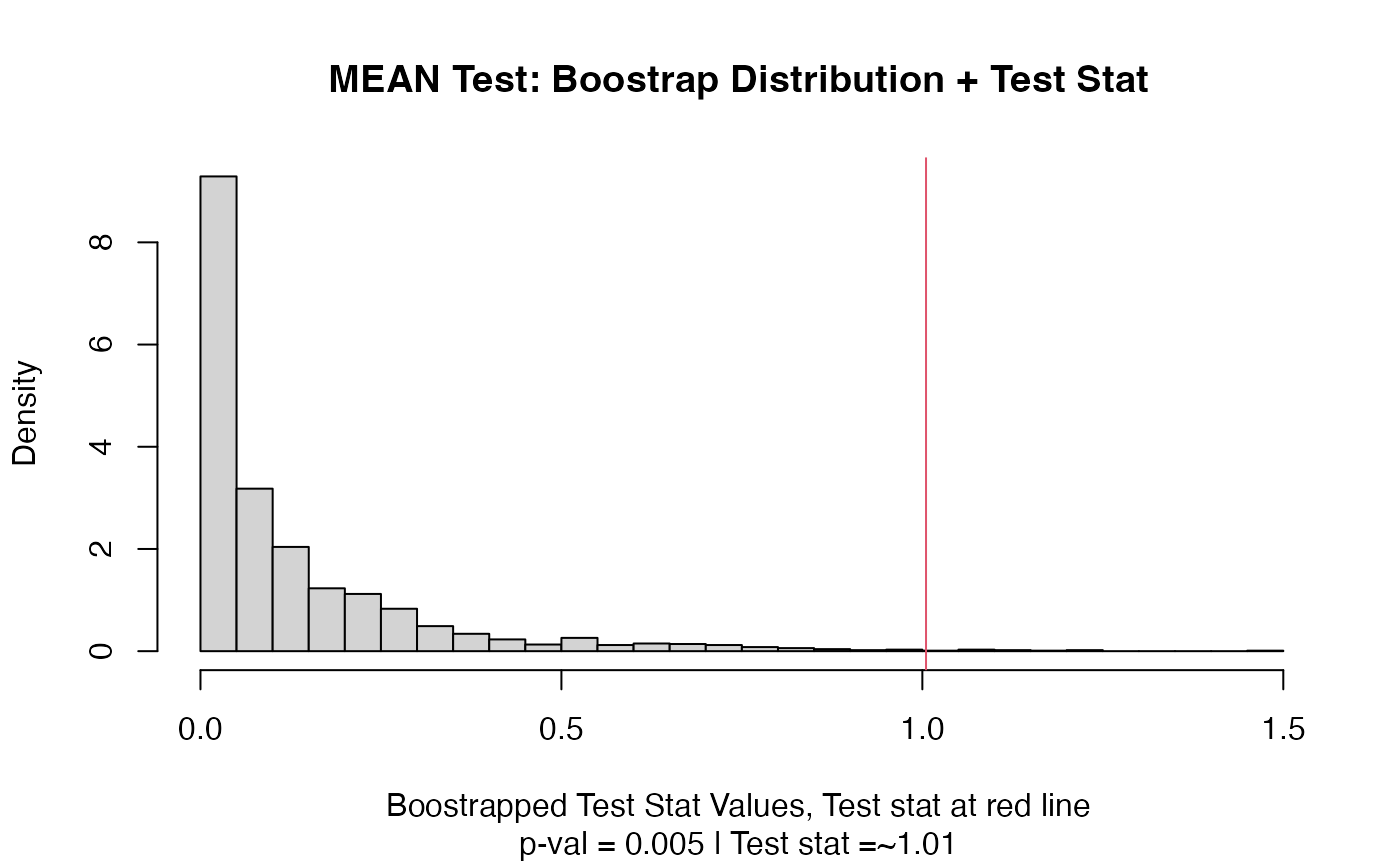(Warning! This function has changed substantially between v1.2.0 and v2.0.0) This function takes a two-sample test statistic and produces a function which performs randomization tests (sampling with replacement) using that test stat. This is an internal function of the twosamples package.

## Usage

permutation_test_builder(test_stat_function, default.p = 2)

## Arguments

test_stat_function

a function of the joint vector and a label vector producing a positive number, intended as the test-statistic to be used.

default.p

This allows for some introduction of defaults and parameters. Typically used to control the power functions raise something to.

## Value

This function returns a function which will perform permutation tests on given test stat.

## Details

test_stat_function must be structured to take two vectors -- the first a combined sample vector and the second a logical vector indicating which sample each value came from, as well as a third and fourth value. i.e. (fun = function(jointvec,labelvec,val1,val2) ...). See examples.

### Conversion Function

Test stat functions designed to work with the prior version of permutation_test_builder will not work. E.g. If your test statistic is

mean_diff_stat = function(x,y,pow) abs(mean(x)-mean(y))^pow

then permutation_test_builder(mean_diff_stat,1) will no longer work as intended, but it will if you run the below code first.

perm_stat_helper = function(stat_fn,def_power) {
output = function(joint,vec_labels,power=def_power,na) {
a = joint[vec_labels]
b = joint[!vec_labels]
stat_fn(a,b,power)
}
output
}

mean_diff_stat = perm_stat_helper(mean_diff_stat)

## Functions

• permutation_test_builder: Takes a test statistic, returns a testing function.

two_sample()

## Examples

mean_stat = function(joint,label,p,na) abs(mean(joint[label])-mean(joint[!label]))**p
myfun = twosamples:::permutation_test_builder(mean_stat,2.0)
set.seed(314159)
vec1 = rnorm(20)
vec2 = rnorm(20,0.5)
out = myfun(vec1,vec2)
out
#> Test Stat   P-Value
#>  1.005096  0.005000
summary(out)
#> MEAN Test
#> =========================
#> Test Statistic: 1.005096
#>        P-Value: 0.005 *
#> - - - - - - - - - - - - -
#>      n1      n2 n.boots
#>      20      20    2000
#> =========================
#> Test stat rejection threshold for alpha = 0.05 is: 0.5232561
#> Null rejected: samples are from different distributions
plot(out)